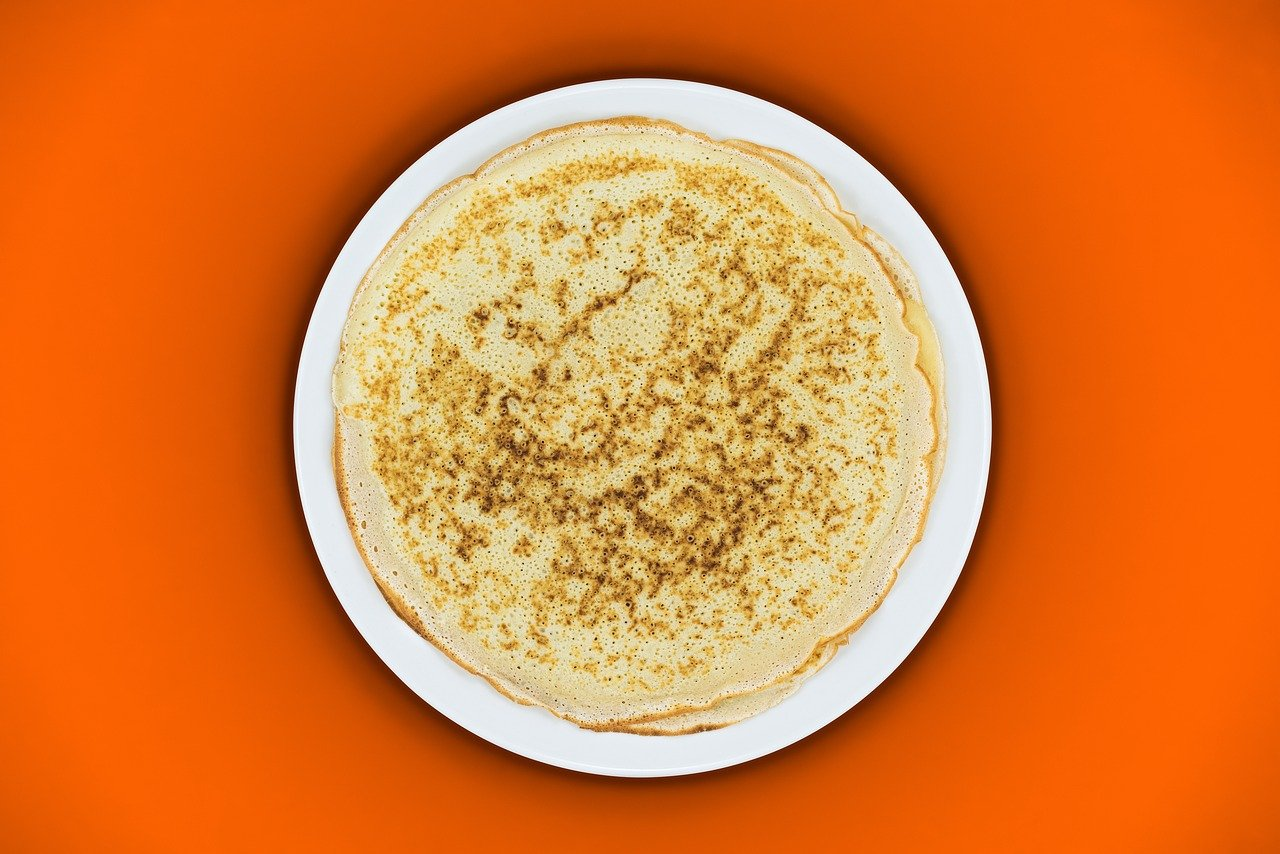# Wrapper classes | Preparing for Java Oracle Associate Certification (OCA) / Java basic technical testsWrapper classes are reference types that allow to manipulate primitives as so. They are thus nullable, and are necessary to store primitive data in collections. Here’s a short overview about specific behaviors of the wrapper classes, including (un)boxing capabilities.

This series of articles are meant for people who already know the basics of Java, that is grammar and vocabulary, and knowledge of OOP. The topics covered correspond to OCA Programmer I level and relate to Java 8 version. If you need to learn the basics, I can recommend the JetBrains Academy Java developer track.

## Wrapper classes

They’re part of the java.lang package, thus need no import to be used.

They use the full type name as their own (for example, wrapper class corresponding to int primitives is Integer).

They implement serializable, extend Number (except Character). The equals() method is implemented (it compares primitive values).

Character implements Serializable and Comparable.

The classes are immutable and final.

There is a wrapper pool, similar to the String pool (explained in a previous post). It only works for wrappers in ranges -128 to 127 (for int/short/byte), char ‘\u0000\’ to ‘\u00ff\’ and boolean values.

### Constructing a wrapper object

A new instance of a wrapper must take a parameter which is the value assigned to it. That’s just logical because they are immutable. Literal numeric values are considered as int, so byte and short values must be explicitly casted, eg new Byte((byte)5); Note that the use of the constructor is deprecated.

A wrapper can also be instantiated by assigning a corresponding primitive value, eg Integer i = 5; , the rule about literal values casting also applies.

On the contrary, you might also do int i = new Wrapper(5);

Note the special behavior of Boolean: new Boolean(“whatever text different than true, ignoring case”) will be considered as false value.

### Wrapper methods

Following methods exist for all primitives*, where xxx is replace by the primitive type name and “Wrapper” represents the corresponding wrapper type:

• xxx xxxValue(Wrapper w) – example: int intValue(myInteger); (instance method)
• xxx Wrapper.parseXxx(String str) – example : int Integer.parseInt(“5”); (static method)
• Wrapper valueOf(xxx p)/(String str) – examples : Integer valueOf(anInt)/(“5”)/(5); (static method) *the method with String parameter doesn’t exist for Character

If the parameter type doesn’t match, an exception is thrown.

Wrappers also hold constants MIN_VALUE and MAX_VALUE returning the minimum and maximum value accepted for the type.

``````int a = 42;
Integer b = Integer.valueOf(a); // thanks to auto boxing, it can just be written Integer b = a;
int c = b.intValue(); // thanks to auto unboxing, it can just be written int c = b;
b = a; // auto unboxing
c = b; // auto boxing
String d = "12.25";
Float e = Float.valueOf(d);
float f = Float.parseFloat(d);
String g = String.valueOf(f);
Double h = Double.valueOf(e);``````

### Auto(un)boxing

A wrapper value transformed into a primitive is called (auto)unboxing, the contrary is (auto)boxing. To remember it, just imagine that a wrap could also be called a box because it contains a primitive.

Autoboxing happens when adding primitive values to collections for example (because collections can only hold reference types, even though it’s not always visible).

Be aware that Java only performs one implicit auto(un)boxing per operation. Thus Integer i = aBytePrimitive; would no work because the byte primitive should virtually be transformed into a Byte, then into an Integer.

``````float i = 2.5f;
double j = 5.3;
Float k = Float.valueOf(j); // doesn't compile
Float l = j; // doesn't compile
Double n = i; // doesn't compile
Long m = a; // doesn't compile
Long o = (long) a;
Long p = Long.valueOf(a);``````

Also be careful as wrappers can hold null values but not primitives.

``````        Float k = null;
float l = k; // compiles but throws null point exception``````

### Comparing wrappers

If using the equals() method on two wrappers of different types, it will always return false.

When the equality operator == is used between a wrapper and a primitive, the primitive value of both is compared.

When the equality operator == is used between two wrappers, the reference is compared!

## Big Decimal

Big Decimal is a special wrapper class. It’s not a OCA topic but well a OCP topic.

Big Decimal is part of the java.math package and has to be imported.

It allows to manipulate decimal numbers with exact precision. Unlike other primitive wrapper (limited by their size), BigDecimal has arbitrary precision.

BigDecimal objects are immutable, “changes” are ignored unless assigned to a variable.

``````BigDecimal bigDecimal = new BigDecimal(5.256995); // unpredictable, for example 0.1 actually equals 0.1000000000000000055511151231257827021181583404541015625
System.out.println(bigDecimal); // outputs 5.256994999999999862438926356844604015350341796875
bigDecimal = bigDecimal.setScale(2, RoundingMode.FLOOR);
System.out.println(bigDecimal); // 5.25

BigDecimal bigDecimal2 = new BigDecimal("5.256995"); // safe way to instantiate a BigDecimal
System.out.println(bigDecimal2); // outputs 5.256995
bigDecimal2 = bigDecimal2.setScale(2, RoundingMode.HALF_UP);
System.out.println(bigDecimal2); // 5.26``````

BigDecimal objects have to be used with arithmetic methods because the operators don’t work with them. Methods take a BigDecimal object as a parameter, return a BigDecimal with the result of the operation and are called:

• subtract
• divide
• multiply
• remainder

They can be chained to avoid intermediate variables.

BigDecimal has an implementation of the methods compareTo and equals. It also has methods to return primitive values (intValue, longValue…) and string values. It also has a few other arithmetic methods you might want to have a look at.

``````BigDecimal bigDecimal = new BigDecimal(5.256995);
BigDecimal bigDecimal2 = new BigDecimal("5.256995");
BigDecimal bigDecimal3 = new BigDecimal("5.256995");
System.out.println(bigDecimal2.equals(bigDecimal)); // false
System.out.println(bigDecimal2.equals(bigDecimal3)); // true
bigDecimal = bigDecimal.setScale(3, RoundingMode.HALF_UP); //5.257
bigDecimal2 = bigDecimal2.setScale(3, RoundingMode.HALF_UP); //5.257
bigDecimal3 = bigDecimal3.setScale(3, RoundingMode.HALF_DOWN); //5.257
System.out.println(bigDecimal2.equals(bigDecimal)); // true
System.out.println(bigDecimal2.equals(bigDecimal3)); // true
bigDecimal2 = bigDecimal2.setScale(2, RoundingMode.CEILING); //5.26
bigDecimal3 = bigDecimal3.setScale(2, RoundingMode.FLOOR); //5.25
System.out.println(bigDecimal2.equals(bigDecimal3)); // false``````